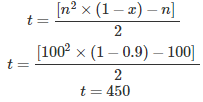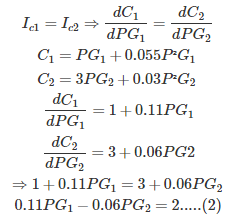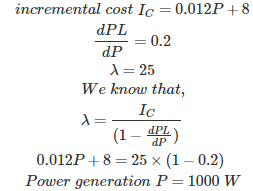# MCQs on Power Systems

##### Page 26 of 67. Go to page 1 2 3 4 5 6 7 8 9 10 11 12 13 14 15 16 17 18 19 20 21 22 23 24 25 26 27 28 29 30 31 32 33 34 35 36 37 38 39 40 41 42 43 44 45 46 47 48 49 50 51 52 53 54 55 56 57 58 59 60 61 62 63 64 65 66 67
01․ Incremental cost of two generators Ic1 = 0.2 P1+60, Ic2 = 0.3 P2+40 and the ratings of the generator are 150 and 250 MW. Find the load sharing of each generator for a load of 200 MW?
P1 = 120 MW, P2 = 80 MW
P1 = 80 MW, P2 = 120 MW
P1 = 100 MW, P2 = 100 MW
P1 = 60 MW, P2 = 140 MW

Given that P1 + P2 = 200 MW Optimum condition of economic load dispatch is Ic1 = Ic2 0.2 P1 + 60 = 0.3 P2 + 40 0.2 P1 - 0.3 P2 = -20 By solving of above equations P1 = 80 MW, P2 = 120 MW

02․ The Y bus matrix of 100 bus interconnected system is 90% sparse. Hence the number of transmission lines in the system must be
250
500
450
900

Total number of transmission lines Where, n = number of buses x = sparsity03․ A power system consists of 300 buses out of which 20 buses are generator buses, 25 buses are the ones with reactive power support buses and 15 buses are ones with fixed shunt capacitors. All the other buses are load buses. It is proposed to perform a load flow analysis for the system using Newton-Raphson Jacobian matrix is
553×553
554×554
555×555
540×540

Size of the Jacobian matrix = (2n-m-2)×(2n-m-2) Where, n = Total number of buses = 300 m = Total number of PV buses m = Voltage control buses + Reactive power support buses + generator buses except slack bus = 44 Fixed shunt capacitors are supplying constant amount of reactive power, so that fixed shunt capacitors are considered as load buses or PQ buses. Size of the Jacobian matrix = (2×300-44-2)×(2×300-44-2) = 554×554

04․ The Gauss Seidel load flow method has following disadvantages. Which of the following statement is wrong?
Unreliable convergence
Slow convergence
Choice of slack bus affects the convergence
A good initial guess for voltages is essential for convergence

Advantages of gauss siedel method: 1. It is a simple algebraical equation, so that calculation time for each iteration is less. 2. More suitable for small size networks. Disadvantages of gauss siedel method: 1. More number of iterations are required, so that it has slow convergence. 2. Initial approximate guessing value is required for convergence. 3. It required accelerating factor for convergence. 4. The choice of slack bus affects the convergence. 5. It is not applicable for the large power system networks.

05․ The incremental fuel costs for two generating units G1 and G2 are given by Ic1 = 25 + 0.2 PG1 and Ic2 = 32 + 0.2 PG2, where PG1 and PG2 are real powers generated by the units. The economic allocation for a total load of 250 MW, neglecting transmission loss, is given by
PG1 = 125 MW and PG2 = 125 MW
PG1 = 142.5 MW and PG2 = 107.5 MW
PG1 = 109.75 MW and PG2 = 140.25 MW
PG1 = 100 MW and PG2 = 150 MW

Given that P1 + P2 = 250 MW Optimum condition of economic load dispatch is Ic1 = Ic2 25 + 0.2 PG1 = 32 + 0.2 PG2 0.2 PG1 - 0.2 PG2 = 7 By solving of above equations PG1 = 142.5 MW and PG2 = 107.5 MW

06․ A lossless power system has to serve a load of 250 MW. There are two generators(G1 and G2) in the system with cost curves C1 and C2 respectively defined as follows: C1 = PG1+0.055P²G1 C2 = 3 PG2 + 0.03 P²G2 Where PG1 and PG2 are the MW injections from generators G1 and G2 respectively. Thus, the minimum cost dispatch will be
PG1 = 0 MW and PG2 = 250 MW
PG1 = 100 MW and PG2 = 150 MW
PG1 = 150 MW and PG2 = 100 MW
PG1 = 250 MW and PG2 = 0 MW

Given that P1 + P2 = 250 MW........(1) Optimum condition of economic load dispatch isAfter solving of above equations PG1 = 100 MW and PG2 = 150 MW

07․ A load centre is at an equidistant from the two thermal generating stations G1 and G2. The fuel cost characteristics of the generating stations are given by F1 = a + bP1 + cP²1 F2 = a + b P2 + 2cP²2 Where P1 and P2 are the generation in MW of G1 and G2, respectively. For most economic generation to meet 300 MW of load, P1 and P2 respectively, are
100, 200
200, 100
150, 150
175, 125

Given that P1 + P2 = 200 MW Optimum condition of economic load dispatch is Ic1 = Ic2 b + 2cP1 = b + 4cP2 2cP1 = 4cP2 P1 = 2P2 Therefore, P1 = 200 MW and P2 = 100 MW

08․ The power generated by two plants are; P1 = 50 MW, P2 = 40 MW. If the loss coefficients are B11 = 0.001, B22 = 0.0025 and B12 = -0.0005, then power loss will be
5.5 MW
8.5 MW
6.5 MW
4.5 MW

Power loss of a transmission line PL = B11×P²1 + B22×P²2 + 2×B12×P1×P2 Where, B11,B22,B12 and B21 are B coefficients P1 and P2 are power delivered by generating stations. Given that, P1 = 50 MW, P2 = 40 MW B11 = 0.001, B22 = 0.0025 and B12 = -0.0005 By substituting the above values, Power loss = 4.5 MW

09․ If, for a given alternator in economic operation mode, the incremental cost is given by (0.012 P + 8) Rs/MWh, dPL/dP = 0.2 and plant λ = 25, then power generation is
1000 MW
1500 MW
1250 MW
750 MW

Given that,10․ The economics of power plant is greatly influenced by